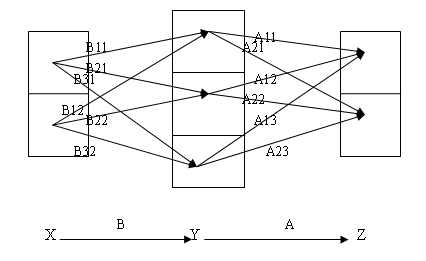# 图示矩阵分块乘法$$AB=\begin{pmatrix}A_{11}&A_{12}&A_{13}\\A_{21}&A_{22}&A_{23}\end{pmatrix}\begin{pmatrix}B_{11}&B_{12}\\B_{21}&B_{22}\\B_{31}&B_{32}\end{pmatrix}$$

$$=\begin{pmatrix}A_{11}B_{11}+A_{12}B_{21}+A_{13}B_{31}&A_{11}B_{12}+A_{12}B_{22}+A_{13}B_{32}\\A_{21}B_{11}+A_{22}B_{21}+A_{23}B_{31}&A_{21}B_{12}+A_{22}B_{22}+A_{23}B_{32}\end{pmatrix}$$

## One thought on “图示矩阵分块乘法”

1. Pingback: 再谈矩阵与矩阵乘法 | 数学笔记